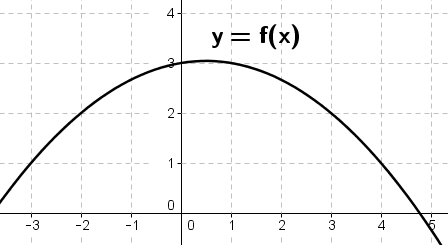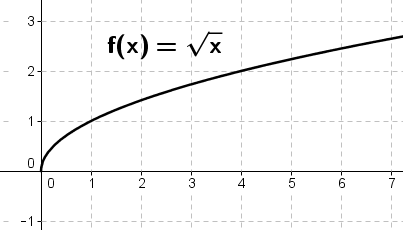## Sction 5.1: Areas

### 1. Divide the interval $$[-2,3]$$ into five subintervals of equal length. Then approximate the area under the curve $$y=f(x)$$ on $$[-2,3]$$ sketching the corresponding rectangles using left endpoints of each subinterval. Repeat this using right end points of each subinterval.solution

solution

### 3. Divide the interval $$[0,4]$$ into four subintervals of equal length. Then approximate the area under the curve $$f(x)=\sqrt{x}$$ on $$[0, 4]$$ sketching the corresponding rectangles using (a) right-end points, and (b) mid-points of each subinterval.solution